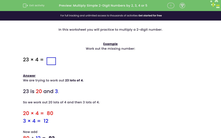# Multiply a Two-digit Number by a One-digit Number Using Partitioning

In this worksheet, students will multiply simple two-digit numbers by a one-digit number.Key stage:  KS 2

Curriculum topic:   Number: Multiplication and Division

Curriculum subtopic:   Write Multiplication/Division Statements for Known Tables

Popular topics:   Place Value worksheets

Difficulty level:#### Worksheet Overview

In this activity, we will be multiplying a two-digit number by a one-digit number.

To do this, we need to partition the two-digit number into its place value.

So, let's try multiplying 22 by 7

The first step is to partition 22.

22 can be partitioned into 20 and 2.

The next step is to multiply the ones and tens by 7.

2 × 7 = 14

20 × 7 = 140

The final step is to add the products together.

140 + 14 = 154

So, 22 × 7 = 154

Let's try an example question together.

Example

Work out the missing number:

23 × 4 =We are trying to work out 23 lots of 4.

23 can be partitioned into 20 and 3.

So we work out 20 lots of 4 and then 3 lots of 4.

20 × 4 =  80

3 × 4 =  12

80 + 12 =  92

So, 23 × 4 =  92

Now you've seen the method to use, it's your turn to try some questions.### What is EdPlace?

We're your National Curriculum aligned online education content provider helping each child succeed in English, maths and science from year 1 to GCSE. With an EdPlace account you’ll be able to track and measure progress, helping each child achieve their best. We build confidence and attainment by personalising each child’s learning at a level that suits them.

Get started#### Popular Maths topics

••••